Processing ......FreeComputerBooks.com Free Computer, Mathematics, Technical Books and Lecture Notes, etc.

Calculus and Mathematical Analysis (Real Analysis, Functional Analysis, etc.)
Related Book Categories:
How many flights will depart from a particular airport? Click here to find out.
•Real Variables with Basic Metric Space Topology (Robert B. Ash)

Designed for a first course in real variables, this text presents the fundamentals for more advanced mathematical work, particularly in the areas of complex variables, measure theory, differential equations, functional analysis, and probability.

•An Introduction to Measure Theory (Terrence Tao)

This is a graduate text introducing the fundamentals of measure theory and integration theory, which is the foundation of modern real analysis, intended to cover a quarter or semester's worth of material for a first graduate course in real analysis.

•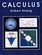Calculus (Gilbert Strang)

This highly regarded calculus textbook is ideal as for self-study. Examples of apps of calculus to physics, engineering and economics are included, as well as many practice questions and illustrative diagrams to assist the reader's grasp of the material.

•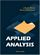Applied Analysis (John K. Hunter, et al)

This book provides an introduction to those parts of analysis that are most useful in applications for graduate students. The material is selected for use in applied problems, and is presented clearly and simply but without sacrificing mathematical rigor.

•Calculus: Theory and Applications (Kenneth Kuttler)

This is a book on single variable calculus including most of the important applications of calculus. It also includes proofs of all theorems presented, either in the text itself, or in an appendix. It also contains an introduction to vectors and vector products.

•Advanced Calculus (Lynn H. Loomis, Shlomo Sternberg)

Problem solving and mathematical modeling are introduced early and reinforced throughout, providing students with a solid foundation in the principles of mathematical thinking.

•Active Calculus (Matt Boelkins)

Rather than detailed explanations and worked out examples, this book uses activities intended to be done by the students in order to present the standard concepts and computational techniques of calculus.

•Introduction to Mathematical Analysis (Beatriz Lafferriere, et al)

The goal of this book is to provide students with a strong foundation in mathematical analysis. Such a foundation is crucial for future study of deeper topics of analysis. It will be reinforced through rigorous proofs.

•Complex Variables: Second Edition (Robert B. Ash, et al)

This text on complex variables is geared toward who have taken an introductory course in real analysis. Offering a concise treatment that provides careful and complete explanations as well as numerous problems and solutions.

•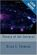Theory of the Integral (Brian S. Thomson)

This text is intended as a treatise for a rigorous course introducing the elements of integration theory on the real line. All of the important features of the Riemann integral, the Lebesgue integral, and the Henstock-Kurzweil integral are covered.

•Complex Integration and Cauchy's Theorem (G.N. Watson)

This brief monograph by one of the great mathematicians of the early 20th century offers a single-volume compilation of propositions employed in proofs of Cauchy's theorem.

•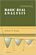Basic Real Analysis (Anthony W. Knapp)

This is a comprehensive treatment with a global view of the Real Analysis, emphasizing the connections between real analysis and other branches of mathematics. Included throughout are many examples and hundreds of problems.

•Introduction to Real Analysis (William F. Trench)

Using an extremely clear and informal approach, this book introduces readers to a rigorous understanding of mathematical analysis and presents challenging math concepts as clearly as possible.

•Integrate Your Brain: How To Do Calculus In Your Head

This book teaches how talented mathematicians utilize their normal, human mental ability to tackle calculus. You are gently trained in the fundamental skills, and shown step by step how to put them into action yourself.

•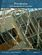Precalculus: An Investigation of Functions (David Lippman, et al)

Problem solving and mathematical modeling are introduced early and reinforced throughout, providing students with a solid foundation in the principles of mathematical thinking.

•Mathematical Analysis I (Elias Zakon)

This book carefully leads the student through the basic topics of real analysis. Topics include metric spaces, open and closed sets, convergent sequences, function limits and continuity, compact sets, sequences and series of functions, power series, etc.

•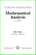Mathematical Analysis II (Elias Zakon)

It is for any second course in real analysis or mathematical analysis, covering Banach spaces, measure theory (with a complete discussion of outer measures, Lebesgue measure, Lebesgue-Stieltjes measures, and differentiation of set functions), etc.

•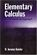Elementary Calculus: An Infinitesimal Approach (H. Jerome Keisler)

This first-year calculus book is centered around the use of infinitesimals. It contains all the ordinary calculus topics, including the basic concepts of the derivative, continuity, and the integral, plus traditional limit concepts and approximation problems.

•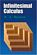Foundations of Infinitesimal Calculus (H. Jerome Keisler)

This monograph is a companion to the Author's textbook. It can be used as a quick introduction to the infinitesimal approach to calculus for mathematicians, as background material for instructors, or as a text for an undergraduate seminar.

•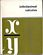Mathematical Background: Foundations of Infinitesimal

We wrote this book of foundations in part to provide a convenient reference for a student who might like to see the "theorem - proof" approach to calculus.

•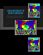Linear Mathematics In Infinite Dimensions (Ulrich H. Gerlach)

Focus on the mathematical framework that underlies linear systems arising in physics, engineering and applied mathematics - from the theory of linear transformation on finite dimensional vector space to the infinite dimensional vector spaces.

•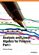Analysis and Linear Algebra for Finance (Patrick Roger)

This book presents the elements of analysis and linear algebra used in financial models and in microeconomics.

•Calculus and Mathematical Analysis

This is the previous page of Calculus and Mathematical Analysis, we are in the processing to convert all the books there to the new page. Please check this page often!!!

Book CategoriesAll CategoriesRecent BooksIT Research LibraryMiscellaneous Books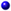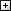Computer LanguagesComputer ScienceData Science/DatabasesElectronic EngineeringJava and Java EE (J2EE)Linux and UnixMathematicsMicrosoft and .NETMobile ComputingNetworking and CommunicationsSoftware EngineeringSpecial TopicsWeb Programming
Other Categories